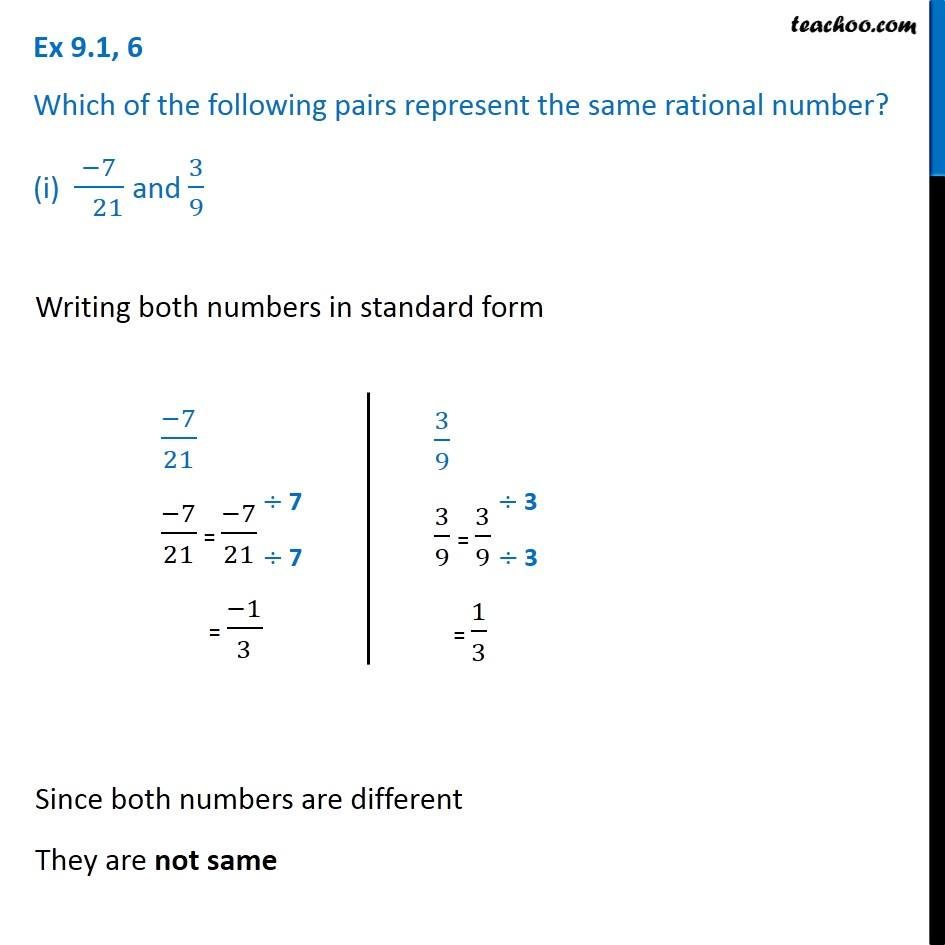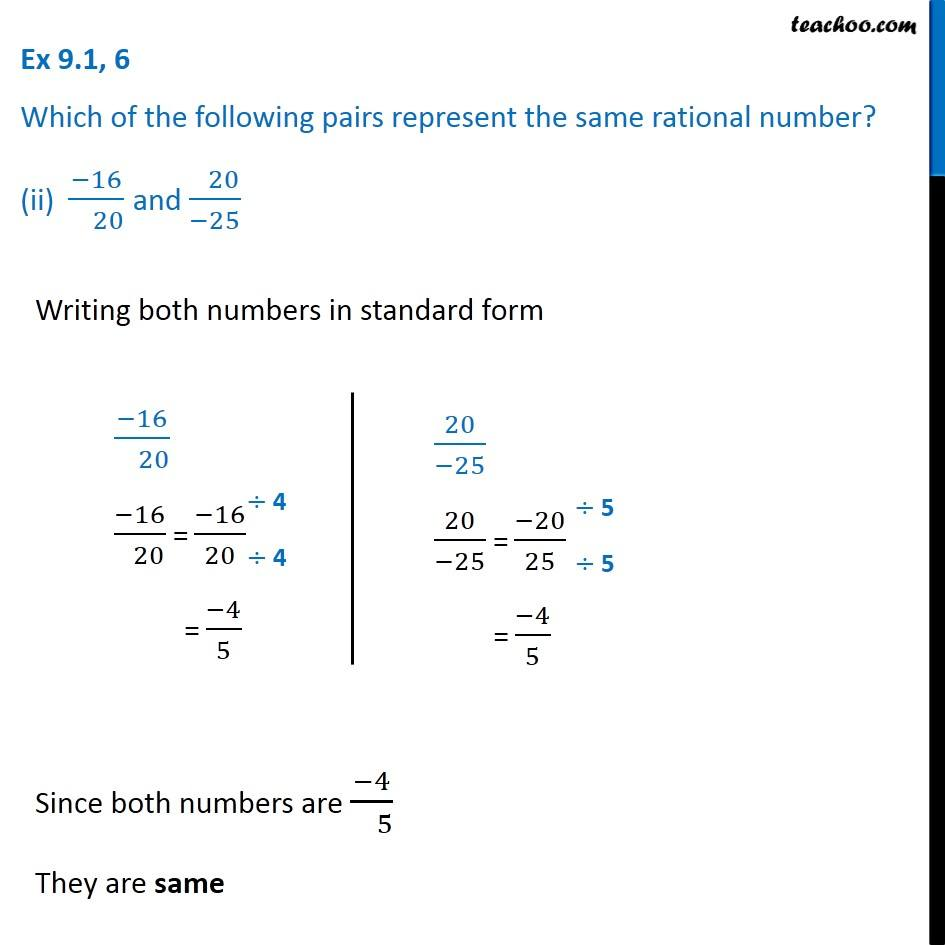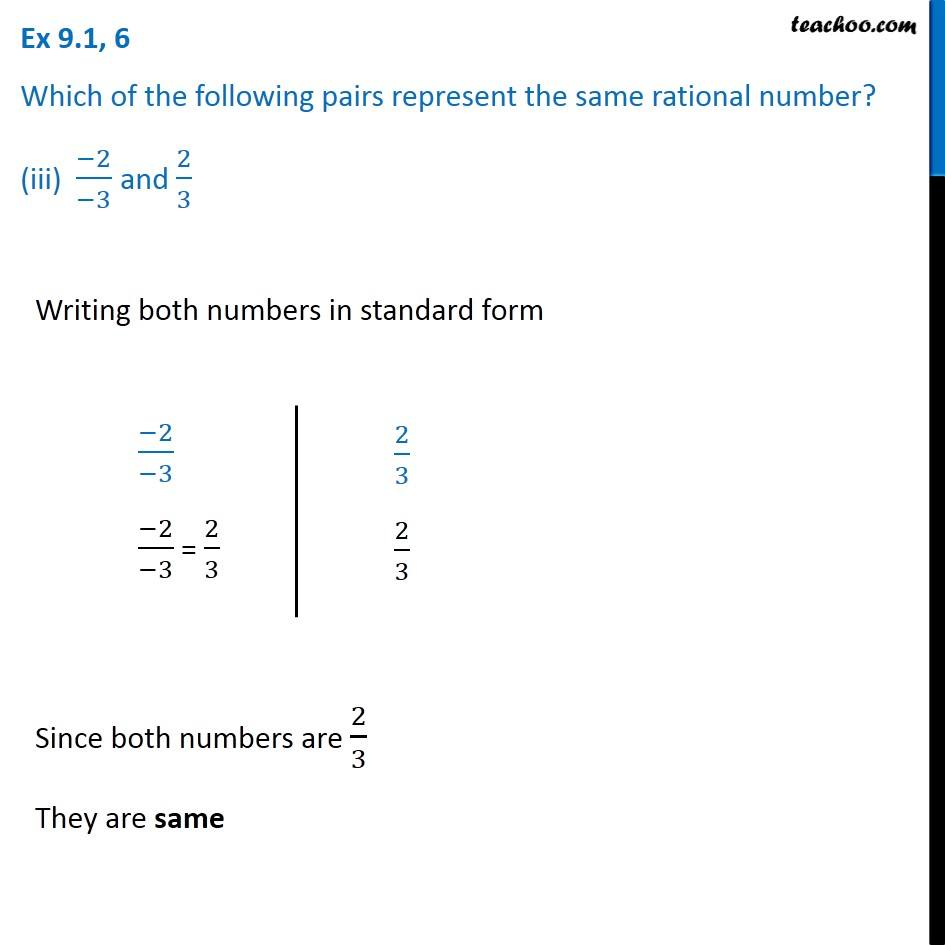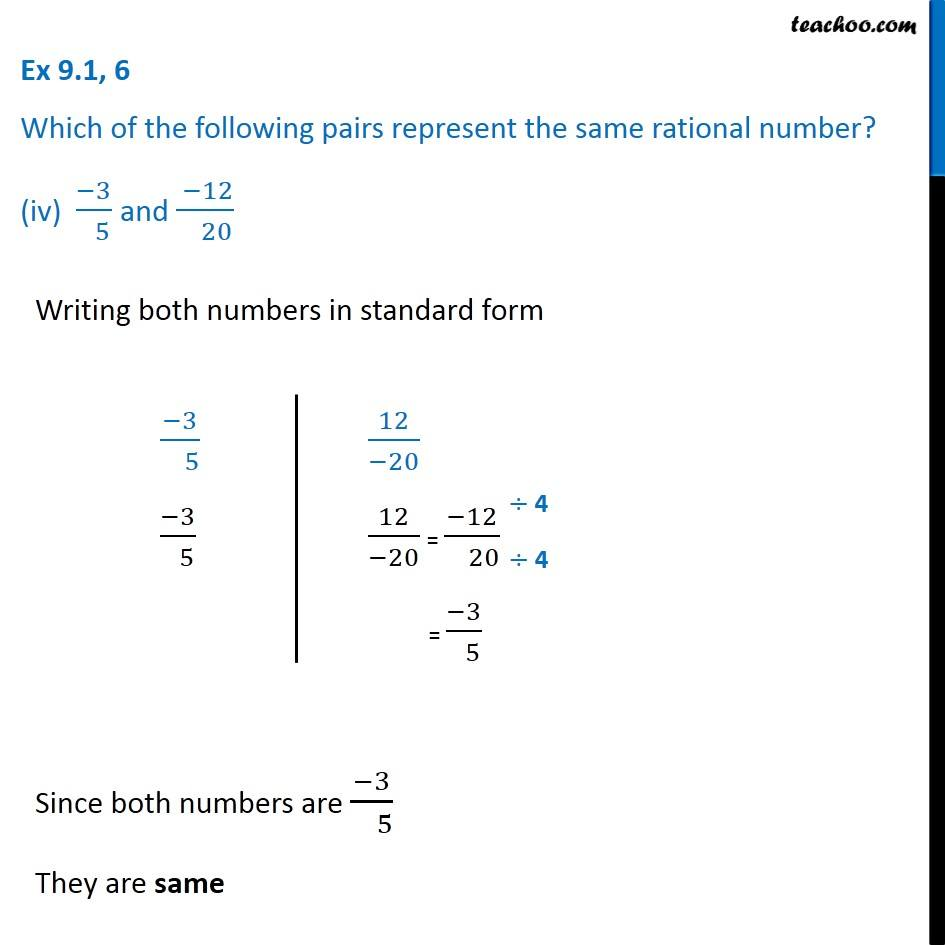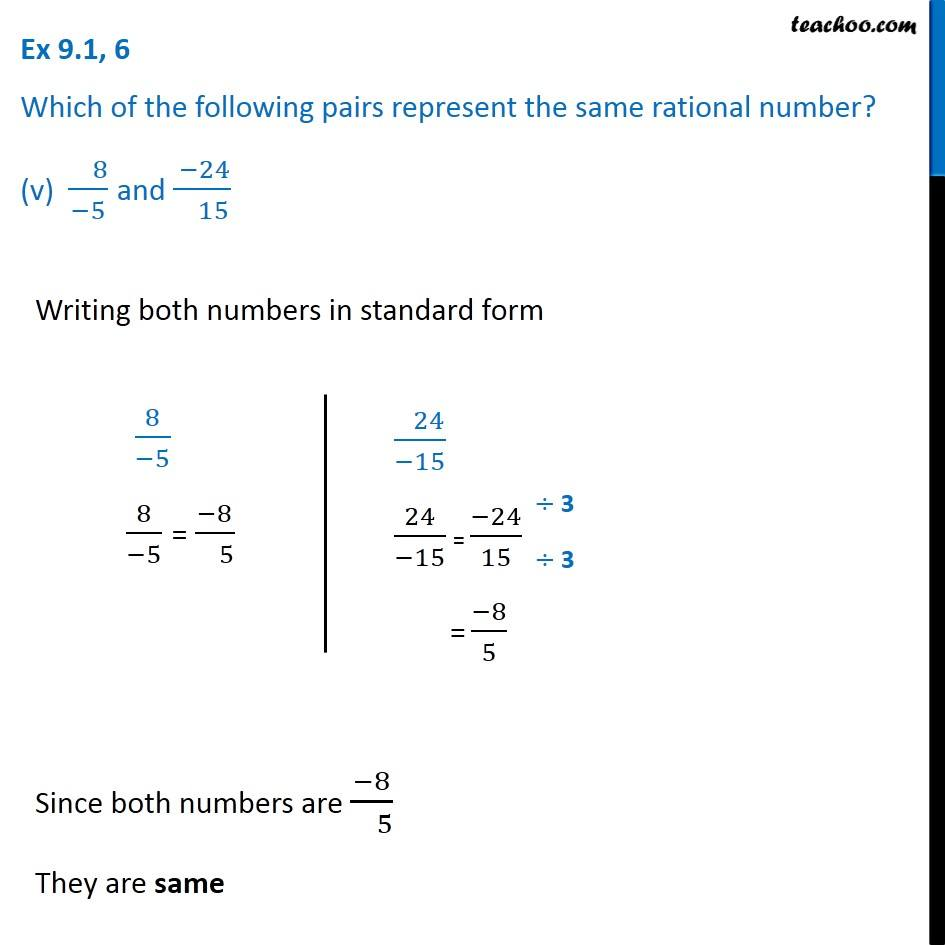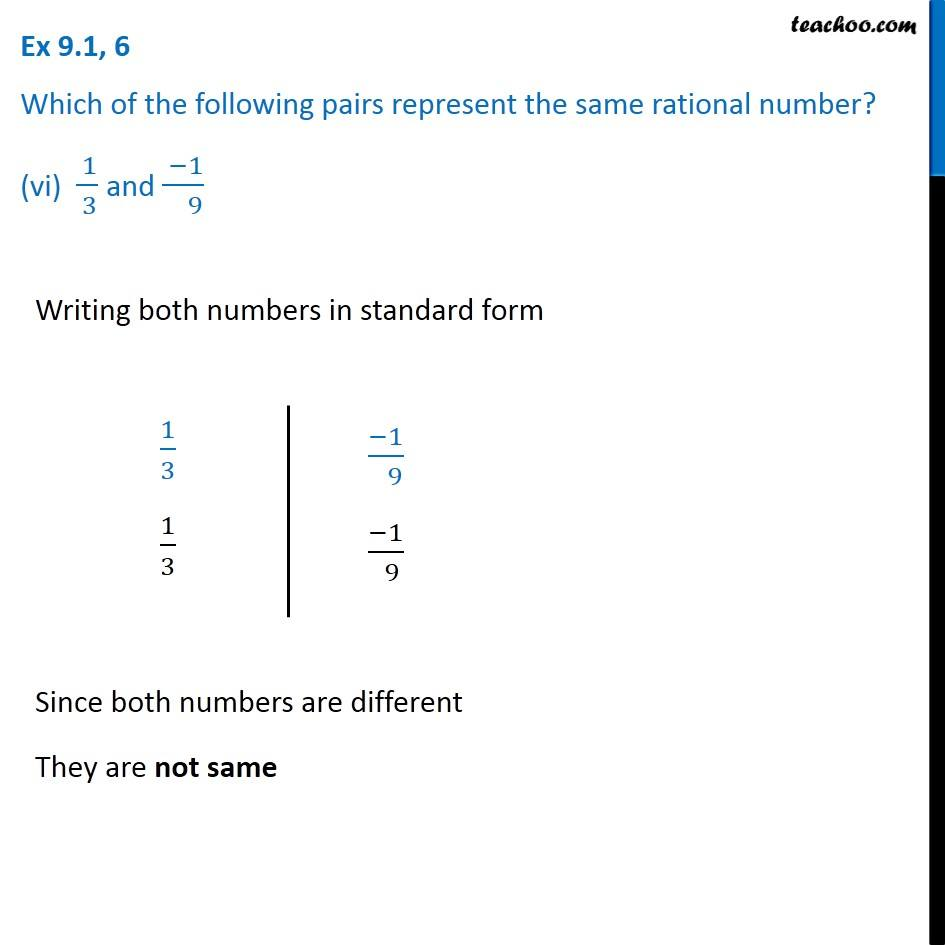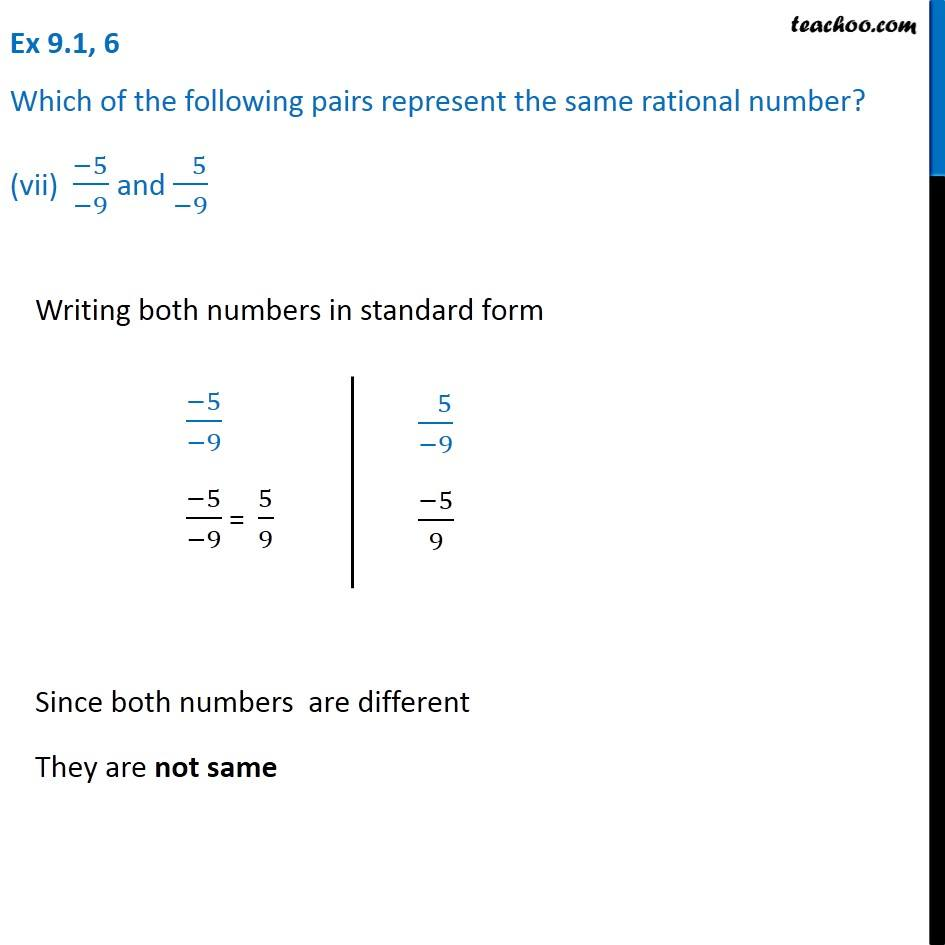1. Chapter 9 Class 7 Rational Numbers
2. Serial order wise
3. Ex 9.1

Transcript

Ex 9.1, 6 Which of the following pairs represent the same rational number? (i) (−7)/( 21) and 3/9 Writing both numbers in standard form (−7)/21 (−7)/21 = (−7)/21 = (−1)/3 3/9 3/9 = 3/9 = 1/3 3/9 3/9 = 3/9 = 1/3 Since both numbers are different They are not same Ex 9.1, 6 Which of the following pairs represent the same rational number? (ii) (−16)/( 20) and ( 20)/(−25) Writing both numbers in standard form (−16)/( 20) (−16)/( 20) = (−16)/20 = (−4)/5 20/(−25) 20/(−25) = (−20)/25 = (−4)/5 Since both numbers are (−4)/( 5) They are same Ex 9.1, 6 Which of the following pairs represent the same rational number? (iii) (−2)/(−3) and 2/3 Writing both numbers in standard form (−2)/(−3) (−2)/(−3) = 2/3 2/3 2/3 Since both numbers are 2/3 They are same Ex 9.1, 6 Which of the following pairs represent the same rational number? (iv) (−3)/( 5) and ( −12)/( 20) Writing both numbers in standard form (−3)/( 5) (−3)/( 5) 12/(−20) 12/(−20) = (−12)/( 20) = (−3)/( 5) Since both numbers are (−3)/( 5) They are same Ex 9.1, 6 Which of the following pairs represent the same rational number? (v) ( 8)/(−5) and ( −24)/( 15) Writing both numbers in standard form 8/(−5) 8/(−5) = (−8)/( 5) ( 24)/(−15) 24/(−15) = (−24)/15 = (−8)/5 Since both numbers are (−8)/( 5) They are same Ex 9.1, 6 Which of the following pairs represent the same rational number? (vi) ( 1)/( 3) and ( −1)/( 9) Writing both numbers in standard form 1/3 1/3 (−1)/( 9) (−1)/( 9) Since both numbers are different They are not same Ex 9.1, 6 Which of the following pairs represent the same rational number? (vii) (−5)/(−9) and ( 5)/(−9) Writing both numbers in standard form (−5)/(−9) (−5)/(−9) = 5/9 ( 5)/(−9) (−5)/9 Since both numbers are different They are not same

Ex 9.1

About the AuthorDavneet Singh
Davneet Singh is a graduate from Indian Institute of Technology, Kanpur. He has been teaching from the past 10 years. He provides courses for Maths and Science at Teachoo.